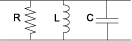shuntRLC

Create two-port shunt RLC circuit

Description

Use the shuntRLC object to create a circuit representing a resistor, inductor, and capacitor connected in parallel or shunt. The shunt RLC circuit object is a two-port network as shown in this circuit diagram.You can also use the shuntRLC object to model a shunt RLC circuit in cascaded stages using the rfbudget object or the RF Budget Analyzer app.

Creation

Description

rlc = shuntRLC creates a default shunt RLC object.

example

rlc = shuntRLC(Name,Value) sets Properties of the shuntRLC object using one or more name-value arguments. For example, rlc = shuntRLC('R',80) creates a shunt RLC object with the resistance set to 80 ohms. Properties you do not specify retain their default values.

Properties

expand all

Name of the shunt RLC circuit, specified as a string scalar or a character vector.

Example: rlc = shuntRLC('Name','RLCshuntcircuit') creates a shunt RLC circuit called RLCshuntcircuit.

Resistance value of the shunt RLC circuit, specified as a positive scalar in ohms.

Example: rlc = shuntRLC('R',75) creates a shunt RLC circuit with the resistance set to 75 ohms.

Inductance value of the shunt RLC circuit, specified as a positive scalar in henries.

Example: rlc = shuntRLC('L',1e-6) creates a shunt RLC circuit with the inductance set to 1e-6 henries.

Capacitance value of the shunt RLC circuit, specified as a positive scalar in farads.

Example: rlc = shuntRLC('C',2.2e-9) creates a shunt RLC circuit with the capacitance set to 2.2e-9 farads.

Number of input and output ports of the shunt RLC circuit, returned as a positive scalar.

Terminals of the shunt RLC circuit, returned as a cell array.

Object Functions

 sparameters Calculate S-parameters for RF data, network, circuit, and matching network objects groupdelay Group delay of S-parameter object or RF filter object or RF Toolbox circuit object noisefigure Calculate noise figure of transmission lines, series RLC, and shunt RLC circuits

Examples

collapse all

Create a shunt RLC circuit with the specified parameters.

rlc = shuntRLC('R',60,'L',1e-3,'C',50e-6);

Calculate the S-parameters of the shunt RLC circuit at 1 GHz.

spar = sparameters(rlc,1e9)
spar =
sparameters: S-parameters object

NumPorts: 2
Frequencies: 1.0000e+09
Parameters: [2x2 double]
Impedance: 50

rfparam(obj,i,j) returns S-parameter Sij

Calculate the noise figure of the shunt RLC circuit at 1 GHz.

nf = noisefigure(rlc,1e9)
nf = 2.6324